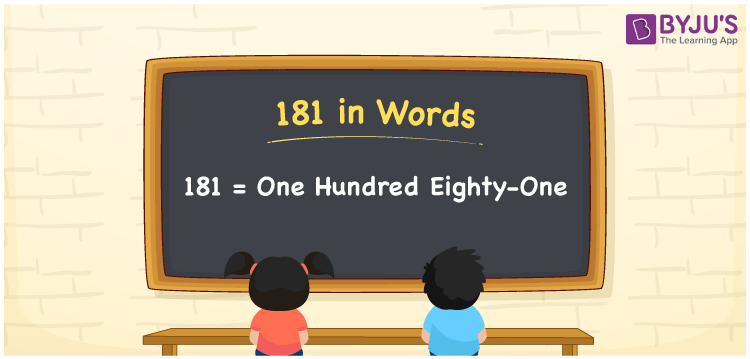# 181 in Words

181 in words is written as One Hundred Eighty-one. The name of number 181 in English is “One Hundred Eighty-one”. The word One Hundred Eighty-one is used in many real-life situations. For example, there are One Hundred Eighty-one students in a classroom. Thus, it is a counting number. Also, to represent the currency equal to 181, we can write it in words as Rupees One Hundred Eighty-one or One Hundred Eighty-one rupees. Therefore, it is necessary to learn the numbers in words, for the ease of understanding and expressing them.

 181 in words One Hundred Eighty-one One Hundred Eighty-one in Numbers 181

## 181 in English Words## How to Write 181 in Words?

If we know the place value of digits of 181, then we can easily express it in words. The place value is basically the position of a digit in a number. 181 is a three-digit number, therefore, we can specify the position of each digit of 181 in a place value chart. In the Indian numbering system, the order of place value of digits from right to left is given by:

 Hundreds Tens Ones 1 8 1

We can write it in expanded form as:

1 × Hundred + 8 × Ten + 1 × One

= 1 × 100 + 8 × 10 + 1 × 1

= 100 + 80 + 1

= 181

= One Hundred Eighty-one

Therefore, 181 in words is written as eight hundred thirties.

181 is a natural number that precedes 182 and succeeds 180.

181 in words – One Hundred Eighty-one

Is 181 an odd number? – Yes

Is 181 an even number? – No

Is 181 a perfect square number? – No

Is 181 a perfect cube number? – No

Is 181 a prime number? – No

Is 181 a composite number? – Yes

## Frequently Asked Questions on 181 in Words

Q1

### How to write 181 in words?

181 in English is written as “One Hundred Eighty-one”.
Q2

### Is 181 an odd number or even number?

181 is an odd number because it is not wholly divisible by 2.
Q3

### Write One Hundred Eighty-one in numbers.

One Hundred Eighty-one in numbers is 181.